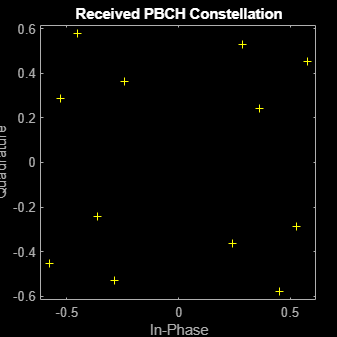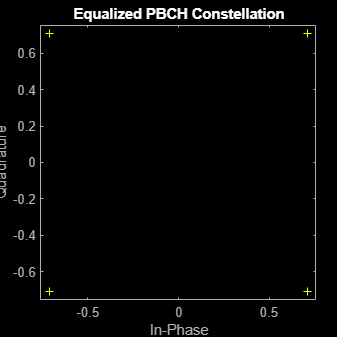# Extract PBCH Symbols and Channel Estimates for Decoding

Extract physical broadcast channel (PBCH) symbols from a received grid and associated channel estimates in preparation for decoding a beamformed PBCH.

### PBCH Coding and Beamforming

Create a random sequence of binary values corresponding to a BCH codeword. The length of the codeword is 864, as specified in TS 38.212 Section 7.1.5. Using the codeword, create symbols and indices for a PBCH transmission. Specify the physical layer cell identity number.

```E = 864; cw = randi([0 1],E,1); ncellid = 17; v = 0; pbchTxSym = nrPBCH(cw,ncellid,v); pbchInd = nrPBCHIndices(ncellid);```

Use `nrExtractResources `to create indices for the two transmit antennas of a beamformed PBCH. Use these indices to map the beamformed PBCH into the transmitter resource array.

```carrier = nrCarrierConfig('NSizeGrid',20); P = 2; txGrid = nrResourceGrid(carrier,P); F = [1 0.3i]; [~,bfInd] = nrExtractResources(pbchInd,txGrid); txGrid(bfInd) = pbchTxSym*F;```

OFDM modulate the PBCH symbols mapped into the transmitter resource array.

`txWaveform = nrOFDMModulate(carrier,txGrid);`

### PBCH Transmission and Decoding

Create and apply a channel matrix to the waveform. Receive the transmitted waveforms.

```R = 3; H = dftmtx(max([P R])); H = H(1:P,1:R); H = H/norm(H); rxWaveform = txWaveform*H;```

Create channel estimates including beamforming.

``` hEstGrid = repmat(permute(H.'*F.',[3 4 1 2]),[240 4]); nEst = 0;```

Demodulate the received waveform using orthogonal frequency division multiplexing (OFDM).

` rxGrid = nrOFDMDemodulate(carrier,rxWaveform);`

In preparation for PBCH decoding, extract symbols from the received grid and the channel estimate grid.

```[pbchRxSym,pbchHestSym] = nrExtractResources(pbchInd,rxGrid,hEstGrid); scatterplot(pbchRxSym(:),[],[],'y+'); title('Received PBCH Constellation');```Equalize the symbols by performing MMSE equalization on the extracted resources. Plot the results.

```pbchEqSym = nrEqualizeMMSE(pbchRxSym,pbchHestSym,nEst); scatterplot(pbchEqSym(:),[],[],'y+'); title('Equalized PBCH Constellation');```Retrieve soft bits by performing PBCH decoding on the equalized symbols.

`pbchBits = nrPBCHDecode(pbchEqSym,ncellid,v)`
```pbchBits = 864×1 1010 × -2.0000 -2.0000 2.0000 -2.0000 -2.0000 2.0000 2.0000 -2.0000 -2.0000 -2.0000 ⋮ ```